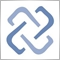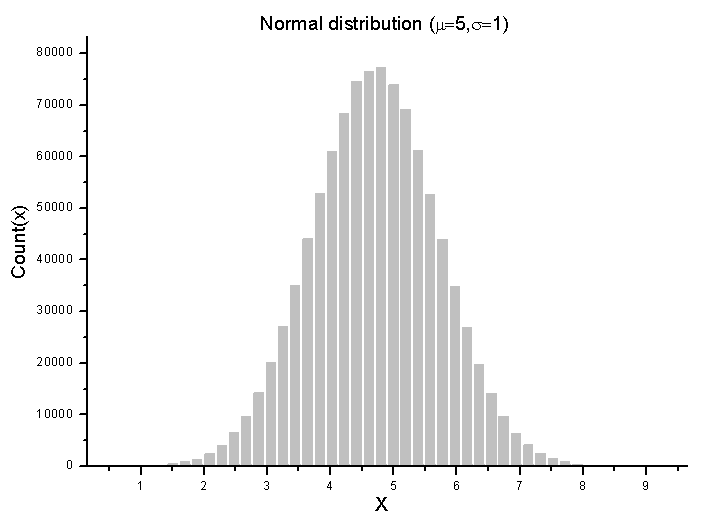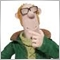# Discussion of article "Statistical Distributions in MQL5 - taking the best of R"Moderator
211590

New article Statistical Distributions in MQL5 - taking the best of R has been published:

The R language is one of the best tools of statistical processing and analysis of data. Thanks to availability and support of multiple statistical distributions, it had become widespread in the analysis and processing of various data. Using the apparatus of probability theory and mathematical statistics allows for a fresh look at the financial market data and provides new opportunities to create trading strategies. With the statistical library, all these features are now available in the MQL5.

Let us consider the functions for working with the basic statistical distributions implemented in the R language.

Those include the Cauchy, Weibull, normal, log-normal, logistic, exponential, uniform, gamma distributions, the central and noncentral beta, chi-squared, Fisher's F-distribution, Student's t-distribution, as well as the discrete binomial and negative binomial distributions, geometric, hypergeometric and Poisson distributions. In addition, there are functions for calculating theoretical moments of distributions, which allow to evaluate the degree of conformity of the real distribution to the modeled one.

The MQL5 standard library has been supplemented with numerous mathematical functions from R. Moreover, an increase in operation speed of 3 to 7 times has been achieved, compared to the initial versions in the R language. At the same time, errors in implementation of certain functions in R have been found.Fig. 2. Distribution histogram of random numbers, generated according to the normal distribution with the parameters mu=5 and sigma=1

Author: MetaQuotes Software Corp.2535
quebralim
Amazing library. Great work!2439

Has anyone used this?